# Subtraction Videos

These math videos demonstrate basic subtraction skills; each video solves an elementary subtraction problem. The videos are intentionally short (under 60 seconds) and demonstrate the solution algorithm while highlighting key points.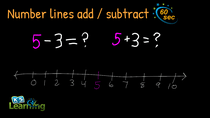Number Lines - Add / Subtract | Explained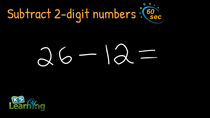Subtract 2-Digit Numbers (No Borrowing) | Example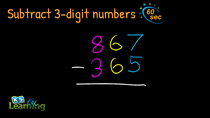Subtract 3-Digit Numbers (No Borrowing) | Example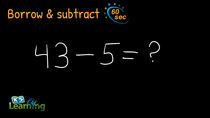Subtraction with Borrowing (Simple) | Explained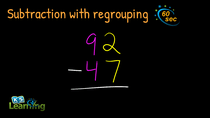Subtraction with Regrouping (2-Digit Numbers) | Example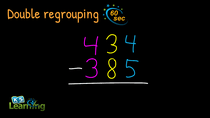Subtraction with Two Regroupings | Example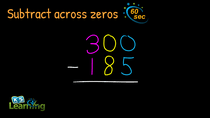Subtracting Across Zeros | Explained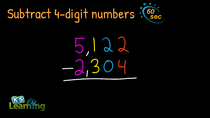Subtract 4-Digit Numbers | Example

What is K5?

K5 Learning offers free worksheets, flashcards and inexpensive workbooks for kids in kindergarten to grade 5. Become a member to access additional content and skip ads.